## Tamilnadu Samacheer Kalvi 12th Maths Solutions Chapter 5 Two Dimensional Analytical Geometry – II Ex 5.5

12th Maths Exercise 5.5 Samacheer Kalvi Question 1.
A bridge has a parabolic arch that is 10m high in the centre and 30m wide at the bottom. Find the height of the arch 6m from the centre, on either sides.
Solution:
From the diagram, equation of the parabolic arch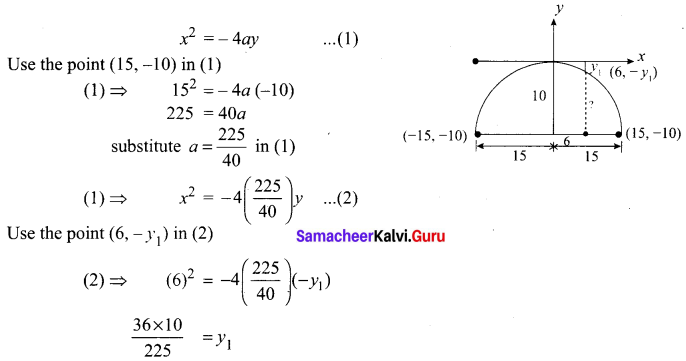∴ The required height =10 – y1 = 10 – 1.6 = 8.4m.

12th Maths Exercise 5.5 Question 2.
A tunnel through a mountain for a four lane highway is to have a elliptical opening. The total width of the highway (not the opening) is to be 16m, and the height at the edge of the road must be sufficient for a truck 4m high to clear if the highest point of the opening is to be 5m approximately. How wide must the opening be?
Solution:
From the diagram,
AA’ = 16 m, OA = 8m, OB = 5m
∴ Equation of the ellipse is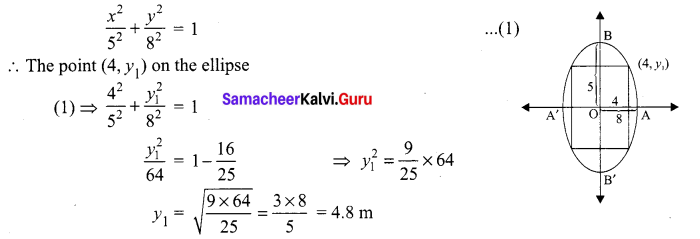∴ The required wide for the opening is 2y1 = 2(4.8) = 9.6 m

12th Maths Exercise 5.2 Samacheer Kalvi Question 3.
At a water fountain, water attains a maximum height of 4m at horizontal distance of 0.5m from its origin. If the path of water is a parabola, find the height of water at a horizontal distance of 0.75m from the point of origin.
Solution:
From the diagram
Equation of the path of water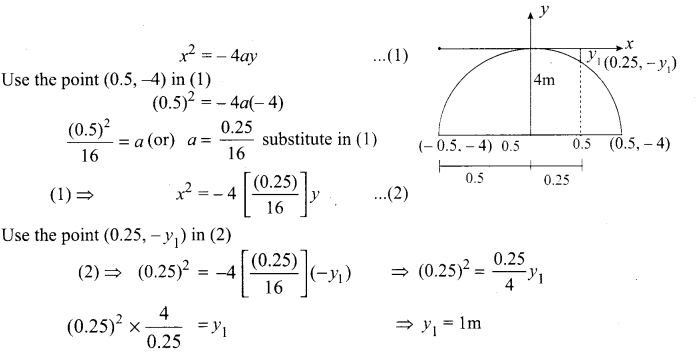The refined height = 4 – y1 = 4 – 1 = 3 m

10th Maths Exercise 5.5 Samacheer Kalvi Question 4.
An engineer designs a satellite dish with a parabolic cross section. The dish is 5m wide at the opening, and the focus is placed 1.2m from the vertex
(a) Position a coordinate system with the origin at the vertex and the x-axis on the parabola’s axis of symmetry and find an equation of the parabola.
(b) Find the depth of the satellite dish at the vertex.
Solution:
From the diagram,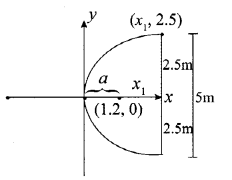(a) Consider the satellite dish is open rightward parabola
y2 = 4 ax ……….. (1)
Clearly a = 1.2m
(1) ⇒ y2 = 4(1.2)
y2 = 4.8x
(b) Use the point (x1, 2.5) in (1)
(2.5)2 = 4(1.2)x1
$$\frac{(2.5)^{2}}{4(1.2)}$$ = y1
x1 = 1.3 m
∴ The depth of the satellite dish at vertex is 1.3 m

Two Dimensional Analytical Geometry Pdf Question 5.
Parabolic cable of a 60m portion of the roadbed of a suspension bridge are positioned as shown below. Vertical Cables are to be spaced every 6m along this portion of the roadbed. Calculate the lengths of first two of these vertical cables from the vertex.
Solution:
From the diagram,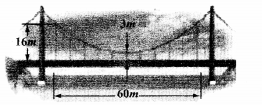Equation of the suspension bridge
(x – h)2 = 4a(y – k)
But V (0, 3)
x2 = 4a (y – 3)
Use the point (30, 16) in (1)
302 = 4a (16 – 3) ⇒ 900 = 13 × 4a
$$\frac{900}{13 \times 4}$$ = a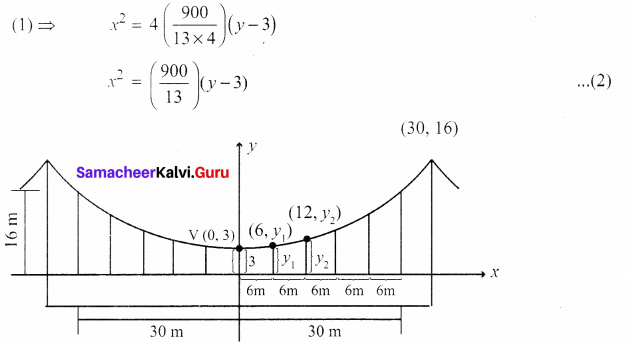(i) The length of the first vertical cable from the vertex is
Use (6, y1) in (2)
(2) ⇒ (6)2 = $$\frac{900}{13}$$ (y1 – 3)
$$\frac{36 \times 13}{900}$$ = y1 – 3
0.52 = y1 – 3
y1 = 3.52 m
(ii) The length of the second vertical cable from the vertex is
Use the point (12, y2) in (2)
(2) ⇒ (12)2 = $$\frac{900}{13}$$ (y2 – 3)
$$\frac{144 \times 13}{900}$$ = y2 – 3
0.52 = y2 – 3
y2 = 3.52 m

12th Samacheer Maths Solutions Question 6.
Cross section of a Nuclear cooling tower is in the shape of a hyperbola with equation $$\frac{x^{2}}{30^{2}}-\frac{y^{2}}{44^{2}}$$ = 1. The tower is 150m tall and the distance from the top of the tower to the centre of the hyperbola is half the distance from the base of the tower to the centre of the hyperbola. Find the diameter of the top and base of the tower.
Solution:
From the diagram,equation of hyperbola is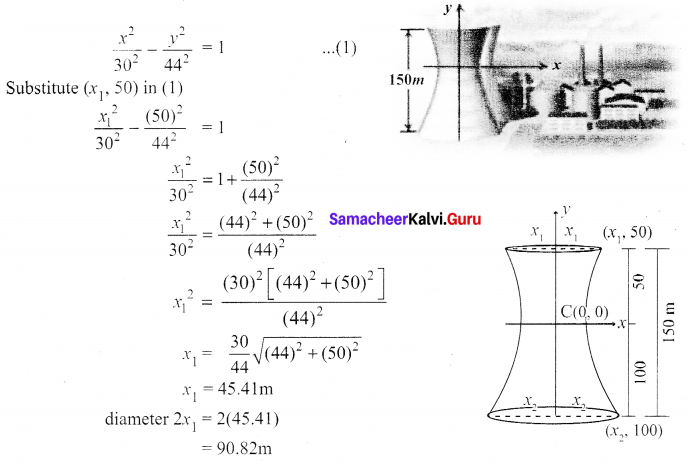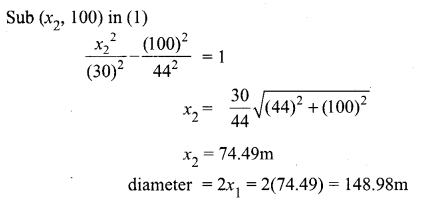Samacheer Kalvi 12th Maths Guide Question 7.
A rod of length 1.2m moves with its ends always touching the coordinate axes. The locus of a point P on the rod, which is 0.3m from the end in contact with x-axis is an ellipse. Find the eccentricity.
Solution:
From the diagram,
(i) ∆le OAB be a right angle triangle.
(ii) ∠APD and ∠PBC are corresponding angles, so corresponding angles are equal.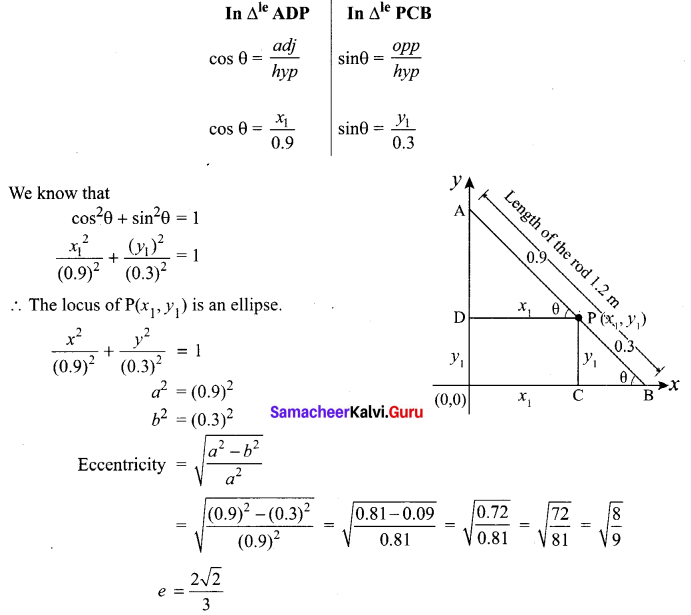Samacheerkalvi.Guru 12th Maths Question 8.
Assume that water issuing from the end of a horizontal pipe, 7.5m above the ground, describes a parabolic path. The vertex of the parabolic path is at the end of the pipe. At a position 2.5m below the line of the pipe, the flow of water has curved outward 3m beyond the vertical line through the end of the pipe. How far beyond this vertical line will the water strike the ground?
Solution:
From the diagram,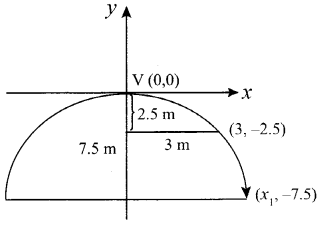Equation of the water path is
x2 = – 4 ay
Use the point (3, – 2.5) in (1)
(3)2 = – 4a(- 2.5)
9 = 10a
a = $$\frac{9}{10}$$ substituting in (1)
(1) ⇒ x2 = -4$$\frac{9}{10}$$y …………. (2)
Use the point (x1, -7.5) in (2)
(2) ⇒ x12 = -4 $$\frac{9}{10}$$(-7.5) ⇒ x12 = 30($$\frac{9}{10}$$)
x1 = $$\sqrt{3 \times 9}$$
x1 = $$3 \sqrt{3}$$ m
∴ The water strikes the ground $$3 \sqrt{3}$$ m beyond the vertical line.

12th Samacheer Maths Solution Question 9.
On lighting a rocket cracker it gets projected in a parabolic path and reaches a maximum height of 4m when it is 6m away from the point of projection. Finally it reaches the ground 12m away from the starting point. Find the angle of projection?
Solution:
From the diagram,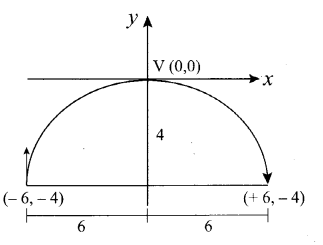Equation of the parabolic path is
x2 = -4ay
Use the point (6, -4) in (1)
(1) ⇒ (6)2 = 16a
$$\frac{36}{16}$$ = 10a
substitute a = $$\frac{9}{14}$$ in (1)
(1) ⇒ x2 = -4$$\left(\frac{9}{4}\right)$$y
x2 = -9y ……….. (2)
Differentiate with respect to ‘x’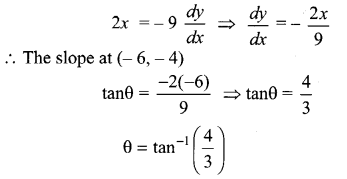12th Maths 5th Chapter In Tamil Question 10.
Points A and B are 10km apart and it is determined from the sound of an explosion heard at those points at different times that the location of the explosion is 6km closer to A than B. Show that the location of the explosion is restricted to a particular curve and find an equation of it.
Solution:
From the diagram,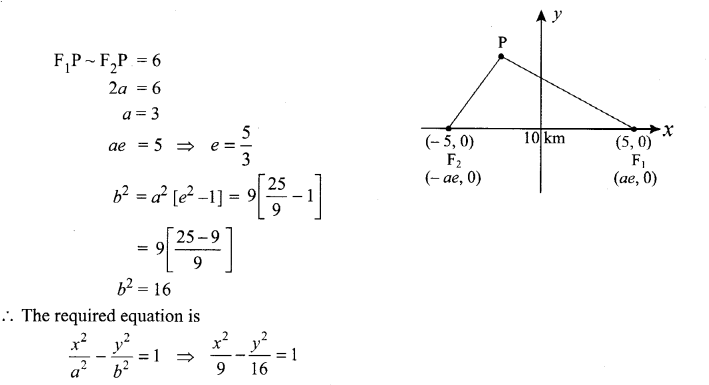### Samacheer Kalvi 12th Maths Solutions Chapter 5 Two Dimensional Analytical Geometry – II Ex 5.5 Additional Problems

Two Dimensional Analytical Geometry 2 Question 1.
If a parabolic reflector is 20cm in diameter and 5cm deep, find the distance of the focus from the centre of the reflector.
Solution: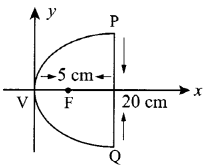By the property of the parabolic reflector, the position of the bulb should be placed at the focus.
By taking the vertex at the origin the equation of the reflector is y2 = 4ax.
Let PQ be the diameter of the reflector P = (5, 10)
Since P (5, 10) lies on the parabola,
102 = 4a × 5
ie., 100 = 20a ⇒ a = 5
So the focus is at a distance of 5cm from the vertex and focus is (5, 0).

Exercise 5.5 Class 12 Question 2.
The focus of a parabolic mirror is at a distance of 8cm from its centre (vertex). If the mirror 25cm deep, find the diameter of the mirror.
Solution: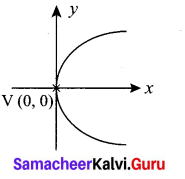Let the vertex be at the origin.
VF = a = 8cm
The equation of the parabola is
Y2 = 4ax = 4(8)x = 32x
Depth of the mirror = x1 = 25cm.
⇒ y2 = 32(25) = 800
y = $$\sqrt{800}=10 \sqrt{8}=10 \times 2 \sqrt{2}=20 \sqrt{2}$$ = Radius of the mirror
∴ Diameter of the mirror = 2 × 20$$\sqrt{2}$$ = 40 $$\sqrt{2}$$ cm of the mirror.

Two Dimensional Analytical Geometry Question 3.
A cable of a suspension bridge is in the form of a parabola whose span is 40 mts. The roadway is 5 mts below the lowest point of the cable. If an extra support is provided across the cable 30 mts above the ground level, find the length of the support if the height of the pillars are 55 mts.
Solution: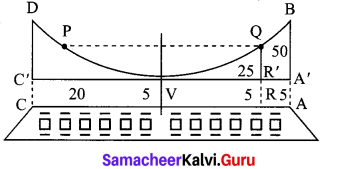The lowest point on the cable is taken as the vertex and it is taken as the origin.
Let AB, CD be the pillars.
Span of parabola = 40 mts = distance between AB and CD
C’V = VA’ = 20 mts
Height of each pillar = 55 mts ⇒ AB = 55 mts
So, A’B = 55 – 5 = 50 mts
Thus, the point B is (20, 50).
Equation of the parabola is x2 = 4ay
Here, B is a point on the parabola, x2 = 4ay
(20)2 = 4a (50) ⇒ 4a = $$\frac{20 \times 20}{50}$$ = 8
∴ The equation is x2 = 8y
Let PQ be the length of the extra support RQ.
RQ = 30, RR’ = 5 ⇒ R’Q = 25
Let VR’ be x1 ∴ Q is (x1, 25).
Q is a point on parabola
x12 = 8 x 25 = 200
x1 = $$\sqrt{200}=10 \sqrt{2}$$
The entire length, PQ = 2 x1 = 20$$\sqrt{2}$$.mts.

Samacheer Kalvi Guru 12th Maths Question 4.
A kho-kho player in a practice session while running realises that the sum of the distances from the kho-kho poles from him is always 8m. Find the equation of the path traced by him if the distance between the poles is 6m.
Solution: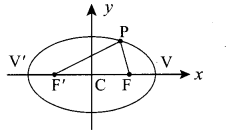Give FP + F’P = 4
ie., 2a = 8 and
FF’ = 2ae = 6
ie., a = 4 and ae = 3
e = $$\frac{a e}{a}=\frac{3}{4}$$
b2 = a2(1 – e2) = 16(1 – $$\frac{9}{16}$$) = 7
So the equation of the path is an ellipse whose equation is $$\frac{x^{2}}{16}+\frac{y^{2}}{7}$$ = 1.